# 10.5 Applications of Parametric Equations

$$\newcommand{\vecs}{\overset { \rightharpoonup} {\mathbf{#1}} }$$ $$\newcommand{\vecd}{\overset{-\!-\!\rightharpoonup}{\vphantom{a}\smash {#1}}}$$$$\newcommand{\id}{\mathrm{id}}$$ $$\newcommand{\Span}{\mathrm{span}}$$ $$\newcommand{\kernel}{\mathrm{null}\,}$$ $$\newcommand{\range}{\mathrm{range}\,}$$ $$\newcommand{\RealPart}{\mathrm{Re}}$$ $$\newcommand{\ImaginaryPart}{\mathrm{Im}}$$ $$\newcommand{\Argument}{\mathrm{Arg}}$$ $$\newcommand{\norm}{\| #1 \|}$$ $$\newcommand{\inner}{\langle #1, #2 \rangle}$$ $$\newcommand{\Span}{\mathrm{span}}$$ $$\newcommand{\id}{\mathrm{id}}$$ $$\newcommand{\Span}{\mathrm{span}}$$ $$\newcommand{\kernel}{\mathrm{null}\,}$$ $$\newcommand{\range}{\mathrm{range}\,}$$ $$\newcommand{\RealPart}{\mathrm{Re}}$$ $$\newcommand{\ImaginaryPart}{\mathrm{Im}}$$ $$\newcommand{\Argument}{\mathrm{Arg}}$$ $$\newcommand{\norm}{\| #1 \|}$$ $$\newcommand{\inner}{\langle #1, #2 \rangle}$$ $$\newcommand{\Span}{\mathrm{span}}$$$$\newcommand{\AA}{\unicode[.8,0]{x212B}}$$

A regular function has the ability to graph the height of an object over time. Parametric equations allow you to actually graph the complete position of an object over time. For example, parametric equations allow you to make a graph that represents the position of a point on a Ferris wheel. All the details like height off the ground, direction, and speed of spin can be modeled using the parametric equations.

What is the position equation and graph of a point on a Ferris wheel that starts at a low point of 6 feet off the ground, spins counterclockwise to a height of 46 feet off the ground, then goes back down to 6 feet in 60 seconds?

## Applying Parametric Equations

There are two types of parametric equations that are typical in real life situations. The first is

circular motion as was described in the concept problem. The second is projectile motion.

Circular Motion

Parametric equations that describe circular motion will have $$x$$ and $$y$$ as periodic functions of sine and cosine. Either $$x$$ will be a sine function and $$y$$ will be a cosine function or the other way around. The best way to come up with parametric equations is to first draw a picture of the circle you are trying to represent.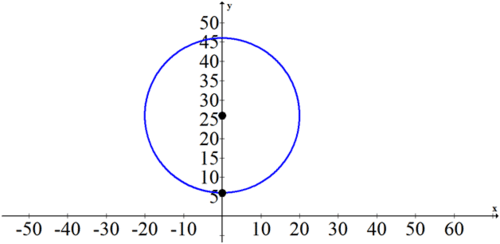Next, it is important to note the starting point, center point and direction. You should already

have the graphs of sine and cosine memorized so that when you see a pattern in words or as a graph, you can identify what you see as $$+\sin ,-\sin ,+\cos ,-\cos$$.

Take the example given above with the Ferris Wheel that starts at a low point of 6 feet off the ground, spins counterclockwise to a height of 46 feet off the ground, then goes back down to 6 feet in 60 seconds. The the vertical component starts at a low point of 6 , travels to a middle point of 26 and then a height of 46 and back down. This is a - cos pattern. The amplitude of the $$-\cos$$ is 20 and the vertical shift is 26 . Lastly, the period is 60 . You can use the period to help you find $$b$$.

\begin{aligned} 60 &=\frac{2 \pi}{b} \\ b &=\frac{\pi}{30} \end{aligned}

Thus the vertical parameterization is:

$$y=-20 \cos \left(\frac{\pi}{30} t\right)+26$$

The horizontal parameterization is found by noticing that the $$x$$ values start at $$0,$$ go up to $$20,$$ go back to 0 , then down to -20 , and finally back to 0 . This is a $$+\sin$$ pattern with amplitude 20. The period is the same as with the vertical component.

Thus parametric equations for the point on the wheel are:

$$x=20 \sin \left(\frac{\pi}{30} t\right)$$

$$y=-20 \cos \left(\frac{\pi}{30} t\right)+26$$

Note that horizontal and vertical components of parametric equations are the $$x=$$ and

$$y=$$ functions respectively or the horizontal and vertical parameterization.

Projectile Motion

Projectile motion has a vertical component that is quadratic and a horizontal component that is linear. This is because there are 3 parameters that influence the position of an object in flight: starting height, initial velocity, and the force of gravity. The horizontal component is independent of the vertical component. This means that the starting horizontal velocity will remain the horizontal velocity for the entire flight of the object.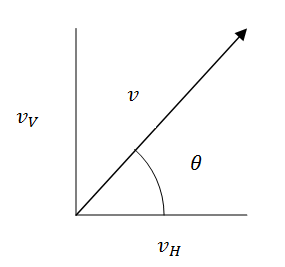Note that gravity, $$g,$$ has a force of about $$-32 \mathrm{ft} / \mathrm{s}^{2}$$ or $$-9.81 \mathrm{~m} / \mathrm{s}^{2}$$. The examples and practice questions in this concept will use feet.

If an object is launched from the origin at a velocity of $$v$$ then it has horizontal and vertical components that can be found using basic trigonometry.

$$\sin \theta=\frac{v_{V}}{v} \rightarrow v \cdot \sin \theta=v_{V}$$

$$\cos \theta=\frac{v_{H}}{v} \rightarrow v \cdot \cos \theta=v_{H}$$

The horizontal component is basically finished. The only adjustments that would have to be made are if the starting location is not at the origin, wind is added or if the projectile travels to the left instead of the right. See Example A.

$$x=t \cdot v \cdot \cos \theta$$

The vertical component also needs to include gravity and the starting height. The general

equation for the vertical component is:

$$y=\frac{1}{2} \cdot g \cdot t^{2}+t \cdot v \cdot \sin \theta+k$$

The constant $$g$$ represents gravity, $$t$$ represents time, $$v$$ represents initial velocity and $$k$$ represents starting height. You will explore this equation further in calculus and physics. Note that in this concept, most answers will be found and confirmed using technology such as your graphing calculator.

## Examples

##### Example 1

A ball is thrown from the point (30,5) at an angle of $$\frac{4 \pi}{9}$$ to the left at an initial velocity of $$68 \mathrm{ft} / \mathrm{s}$$. Model the position of the ball over time using parametric equations. Use your graphing calculator to graph your equations for the first four seconds while the ball is in the air.

The horizontal component is $$x=-t \cdot 68 \cdot \cos \left(\frac{4 \pi}{9}\right)+30$$. Note the negative sign because the object is traveling to the left and the +30 because the object starts at (30,5).

The vertical component is $$y=\frac{1}{2} \cdot(-32) \cdot t^{2}+t \cdot 68 \cdot \sin \left(\frac{4 \pi}{9}\right)+5$$. Note that $$g=-32$$ because gravity has a force of $$-32 \mathrm{ft} / \mathrm{s}^{2}$$ and the +5 because the object starts at (30,5).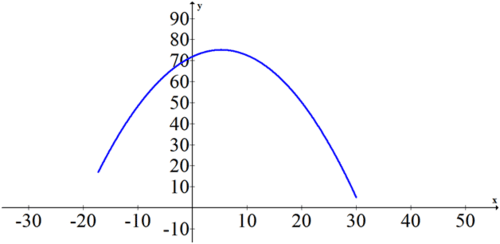##### Example 2

When does the ball from Example 1 reach its maximum and when does the ball hit the ground? How far did the person throw the ball? T

o find when the function reaches its maximum, you can find the vertex of the parabola. Analytically this is messy because of the decimal coefficients in the quadratic. Use your calculator to approximate the maximum after you have graphed it. Depending on how small you make your $$T_{\text {step }}$$ should find the maximum height to be about 75 feet.

To find out when the ball hits the ground, you can set the vertical component equal to zero and solve the quadratic equation. You can also use the table feature on your calculator to determine when the graph goes from having a positive vertical value to a negative vertical value. The benefit for using the table is that it simultaneously tells you the $$x$$ value of the zero.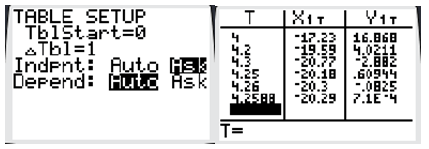After about 4.2588 seconds the ball hits the ground at (-20.29, 0). This means the person threw the ball from (30, 5) to (-20.29, 0), a horizontal distance of just over 50 feet.

##### Example 3

Kieran is on a Ferris wheel and his position is modeled by the parametric equations:

$$x_{K}=10 \cdot \cos \left(\frac{\pi}{5} t\right)$$

$$y_{K}=10 \cdot \sin \left(\frac{\pi}{5} t\right)+65$$

Jason throws the ball modeled by the equation in Example 1 towards Kieran who can catch the ball if it gets within three feet. Does Kieran catch the ball?

This question is designed to demonstrate the power of your calculator. If you simply model the two equations simultaneously and ignore time you will see several points of intersection. This graph is shown below on the left. These intersection points are not interesting because they represent where Kieran and the ball are at the same place but at different moments in time.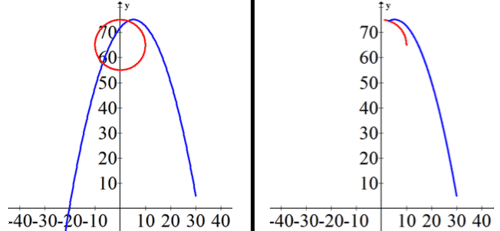When the $$T_{m a x}$$ is adjusted to 2.3 so that each graph represents the time from 0 to 2.3 , you get a better sense that at about 2.3 seconds the two points are close. This graph is shown above on the right.

You can now use your calculator to help you determine if the distance between Kieran and the ball actually does go below 3 feet. Start by plotting the ball's position in your calculator as

$$x_{1}$$ and $$y_{1}$$ and Kieran's position as $$x_{2}$$ and $$y_{2}$$. Then, plot a new parametric equation that compares the distance between these two points over time. You can put this under $$x_{3}$$ and $$y_{3}$$. A calculator can reference internal variables like $$x_{1}, y_{1}$$ that have already been set in the calculator's memory to form new variables like $$x_{3}, y_{3}$$. Note that you can find the $$x_{1}, x_{2}, y_{1}, y_{2}$$ entries in the vars and parametric menu.

$$x_{3}=t$$

$$y_{3}=\sqrt{\left(x_{2}-x_{1}\right)^{2}+\left(y_{2}-y_{1}\right)^{2}}$$

Now when you graph, you should change your window settings and let $$t$$ vary between 0 and $$4,$$ the $$x$$ window show between 0 and 4 and the $$y$$ window show between 0 and $$5 .$$ This way it should be clear if the distance truly does get below 3 feet.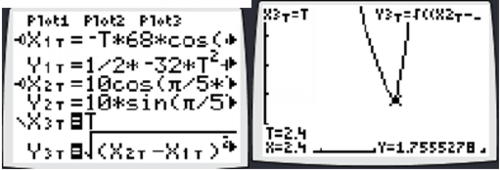Depending on how accurate your $$T_{\text {step }}$$ is, you should find that the distance is below 3 feet. Kieran does indeed catch the ball.

##### Example 4

At what velocity does a football need to be thrown at a $$45^{\circ}$$ angle in order to make it all the way across a football field?

A football field is 100 yards or 300 feet. The parametric equations for a football thrown from (300,0) back to the origin at speed $$v$$ are:

$$x=-t \cdot v \cdot \cos \left(\frac{\pi}{4}\right)+300$$

$$y=\frac{1}{2} \cdot(-32) \cdot t^{2}+t \cdot v \cdot \sin \left(\frac{\pi}{4}\right)$$

Substituting the point (0,0) in for $$(x, y)$$ produces a system of two equations with two variables $$v, t$$

$$0=-t \cdot v \cdot \cos \left(\frac{\pi}{4}\right)+300$$

$$0=\frac{1}{2} \cdot(-32) \cdot t^{2}+t \cdot v \cdot \sin \left(\frac{\pi}{4}\right)$$

You can solve this system many different ways.

$$t=\frac{5 \sqrt{3}}{2} \approx 4.3$$ seconds, $$v=40 \sqrt{6} \approx 97.98 \mathrm{ft} / \mathrm{s}$$

In order for someone to throw a football at a $$45^{\circ}$$ angle all the way across a football field, they would need to throw at about $$98 \mathrm{ft} / \mathrm{s}$$ which is about $$66.8 \mathrm{mph}$$.

$$\frac{98 \text { feet }}{1 \text { sec }} \cdot \frac{3600 \text { sec }}{1 \text { hour }} \cdot \frac{1 \text { mile }}{5280 \text { feet }} \approx \frac{66.8 \text { miles }}{1 \text { hour }}$$

##### Example 5

Nikki got on a Ferris wheel ten seconds ago. She started 2 feet off the ground at the lowest point of the wheel and will make a complete cycle in four minutes. The ride reaches a maximum height of 98 feet and spins clockwise. Write parametric equations that model Nikki’s position over time. Where will Nikki be three minutes from now?

Don't let the 10 second difference confuse you. In order to deal with the time difference, use $$\left(t+\frac{1}{6}\right)$$ instead of $$t$$ in each equation. When $$t=0,$$ ten seconds $$\left(\frac{1}{6}\right.$$ of a minute $$)$$ have already elapsed.

$$x=-48 \cdot \sin \left(\frac{\pi}{2}\left(t+\frac{1}{6}\right)\right)$$

$$y=-48 \cdot \cos \left(\frac{\pi}{2}\left(t+\frac{1}{6}\right)\right)+50$$

At $$t=3, x \approx 46.36$$ and $$y \approx 37.58$$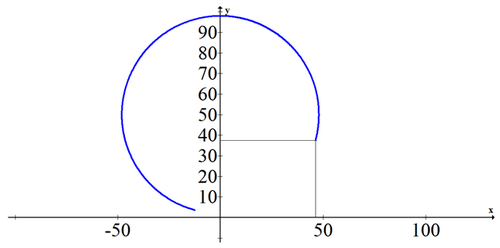##### Review

Candice gets on a Ferris wheel at its lowest point, 3 feet off the ground. The Ferris wheel spins

clockwise to a maximum height of 103 feet, making a complete cycle in 5 minutes.

1. Write a set of parametric equations to model Candice's position.

2. Where will Candice be in two minutes?

3. Where will Candice be in four minutes?

One minute ago Guillermo got on a Ferris wheel at its lowest point, 3 feet off the ground. The

Ferris wheel spins clockwise to a maximum height of 83 feet, making a complete cycle in 6

minutes.

4. Write a set of parametric equations to model Guillermo's position.

5. Where will Guillermo be in two minutes?

6. Where will Guillermo be in four minutes?

Kim throws a ball from (0,5) to the right at 50 mph at a $$45^{\circ}$$ angle.

7. Write a set of parametric equations to model the position of the ball.

8. Where will the ball be in 2 seconds?

9. How far does the ball get before it lands?

David throws a ball from (0,7) to the right at 70 mph at a $$60^{\circ}$$ angle. There is a 6 mph wind in

David's favor.

10. Write a set of parametric equations to model the position of the ball.

11. Where will the ball be in 2 seconds?

12. How far does the ball get before it lands?

Suppose Riley stands at the point (250,0) and launches a football at 72 mph at an angle of $$60^{\circ}$$

towards Kristy who is at the origin. Suppose Kristy also throws a football towards Riley at 65

mph at an angle of $$45^{\circ}$$ at the exact same moment. There is a 6 mph breeze in Kristy's favor.

13. Write a set of parametric equations to model the position of Riley's ball.

14. Write a set of parametric equations to model the position of Kristy's ball.

15. Graph both functions and explain how you know that the footballs don't collide even though the two graphs intersect.

This page titled 10.5 Applications of Parametric Equations is shared under a CK-12 license and was authored, remixed, and/or curated by CK-12 Foundation via source content that was edited to the style and standards of the LibreTexts platform; a detailed edit history is available upon request.